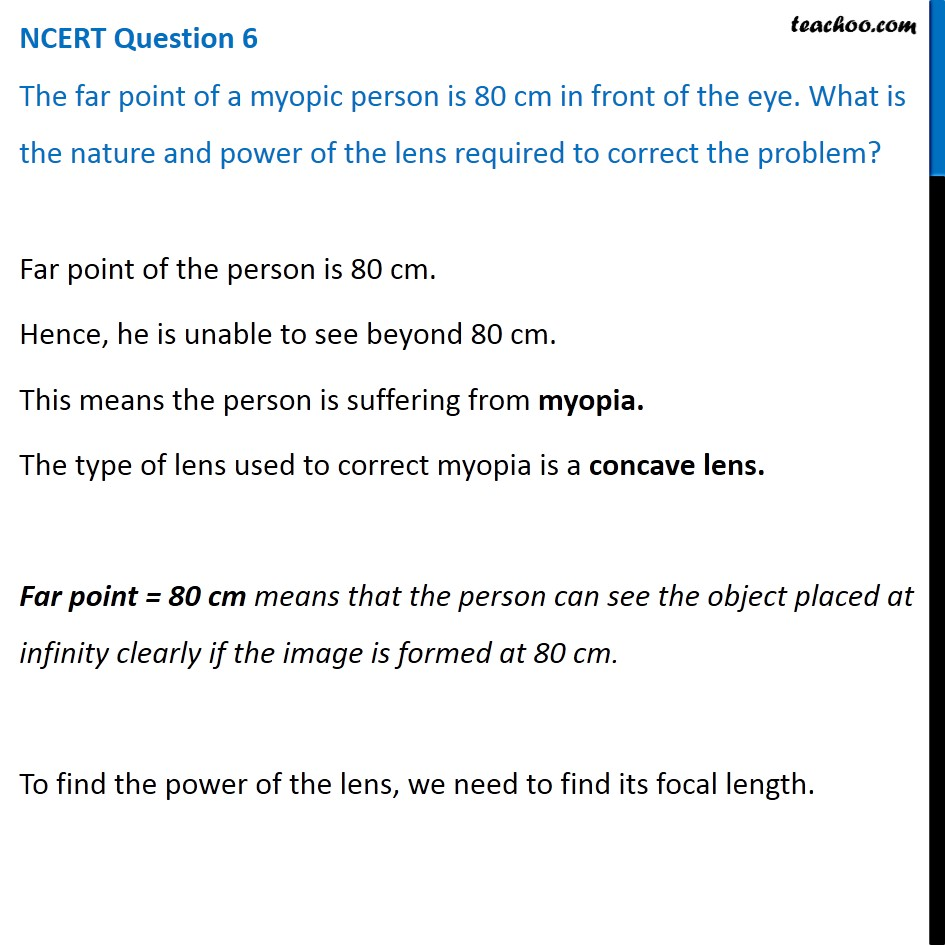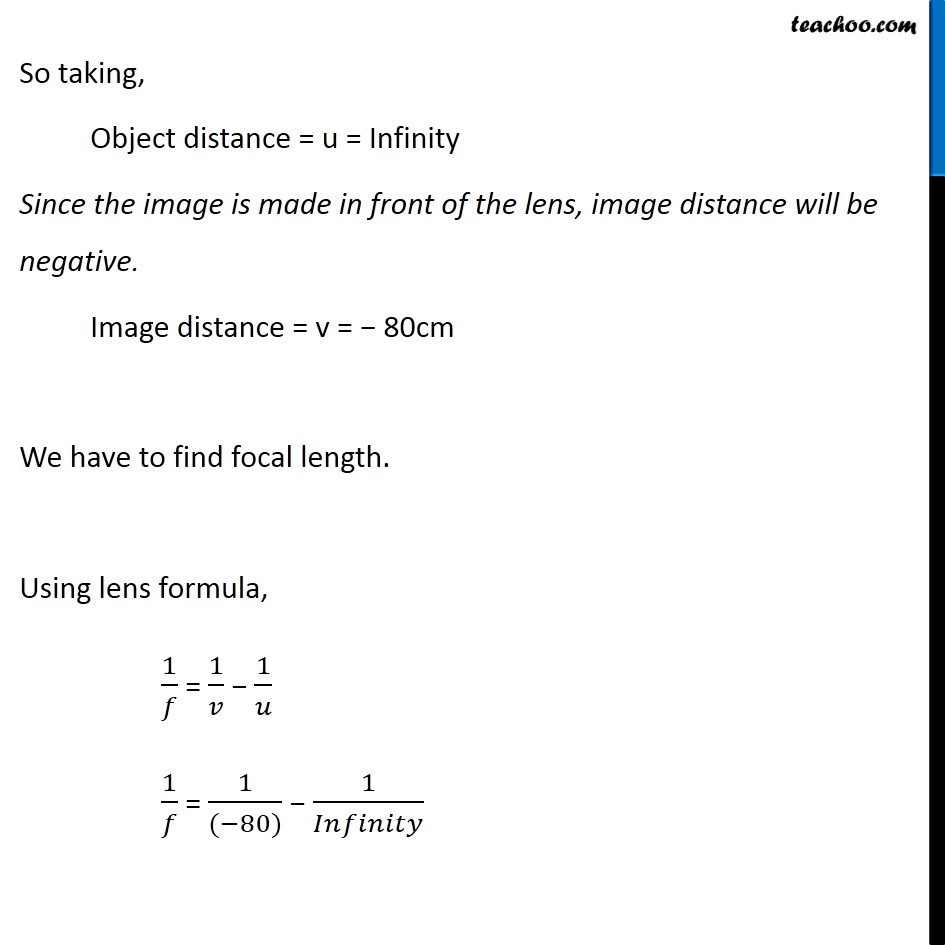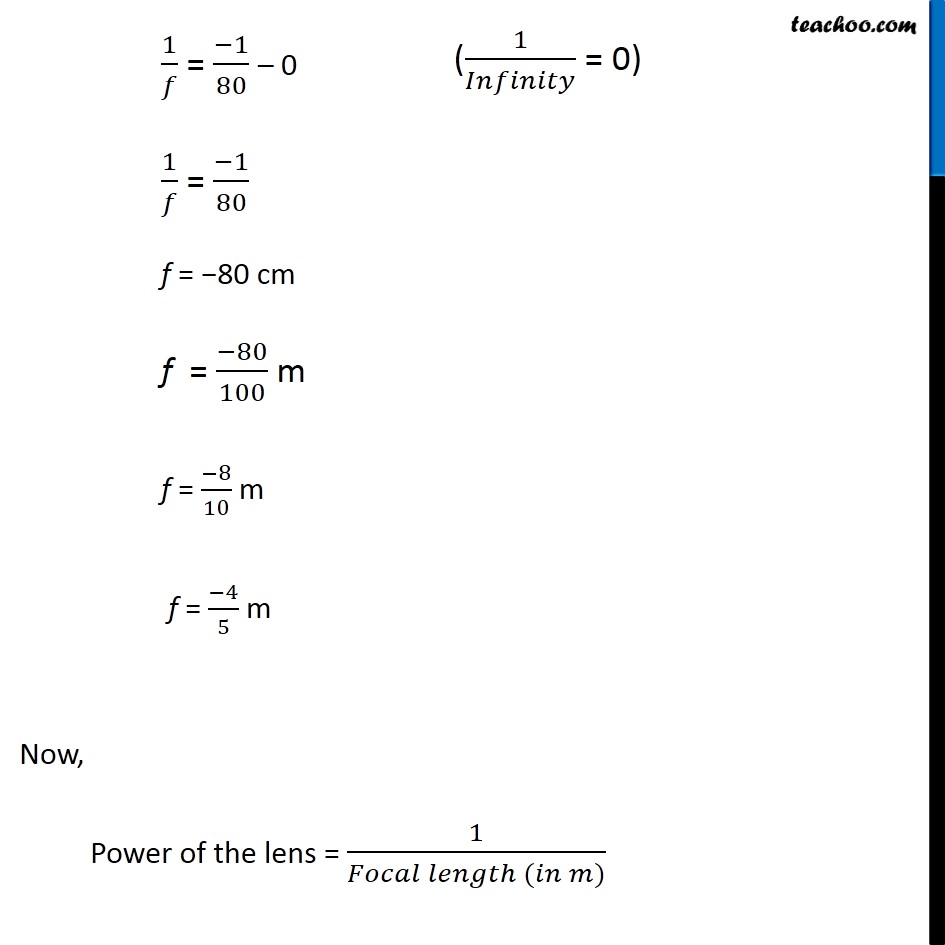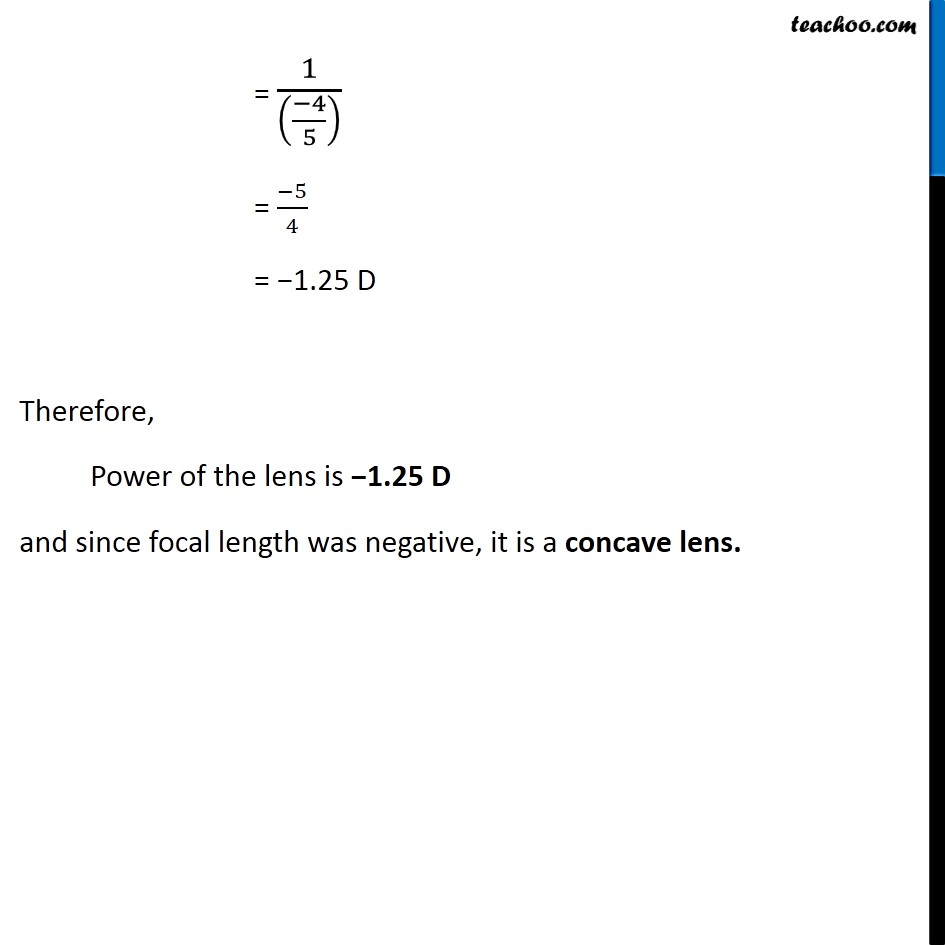NCERT Questions

Class 10
Chapter 11 Class 10 - Human Eye and Colourful World

## The far point of a myopic person is 80 cm in front of the eye. What is the nature and power of the lens required to correct the problem?Learn in your speed, with individual attention - Teachoo Maths 1-on-1 Class

### Transcript

NCERT Question 6 The far point of a myopic person is 80 cm in front of the eye. What is the nature and power of the lens required to correct the problem? Far point of the person is 80 cm. Hence, he is unable to see beyond 80 cm. This means the person is suffering from myopia. The type of lens used to correct myopia is a concave lens. Far point = 80 cm means that the person can see the object placed at infinity clearly if the image is formed at 80 cm. To find the power of the lens, we need to find its focal length. So taking, Object distance = u = Infinity Since the image is made in front of the lens, image distance will be negative. Image distance = v = − 80cm We have to find focal length. Using lens formula, 1/𝑓 = 1/𝑣 − 1/𝑢 1/𝑓 = 1/((−80)) − 1/𝐼𝑛𝑓𝑖𝑛𝑖𝑡𝑦 1/𝑓 = (−1)/80 – 0 1/𝑓 = (−1)/80 f = −80 cm f = (−80)/100 m f = (−8)/10 m f = (−4)/5 m Now, Power of the lens = 1/(𝐹𝑜𝑐𝑎𝑙 𝑙𝑒𝑛𝑔𝑡ℎ (𝑖𝑛 𝑚)) = 1/(((−4)/5) ) = (−5)/4 = −1.25 D Therefore, Power of the lens is −1.25 D and since focal length was negative, it is a concave lens. = 1/(((−4)/5) ) = (−5)/4 = −1.25 D Therefore, Power of the lens is −1.25 D and since focal length was negative, it is a concave lens.